# Diagram#

class discopy.rigid.Diagram(inside, dom, cod, _scan=True)[source]#

A rigid diagram is a closed diagram with `Cup` and `Cap` boxes.

Parameters:
• inside (tuple[Layer, ...]) – The layers of the diagram.

• dom (Ty) – The domain of the diagram, i.e. its input.

• cod (Ty) – The codomain of the diagram, i.e. its output.

Example

```>>> I, n, s = Ty(), Ty('n'), Ty('s')
>>> Alice, jokes = Box('Alice', I, n), Box('jokes', I, n.r @ s)
>>> d = Alice >> Id(n) @ jokes >> Cup(n, n.r) @ Id(s)
>>> d.draw(figsize=(3, 2),
...        path='docs/_static/rigid/diagram-example.png')
```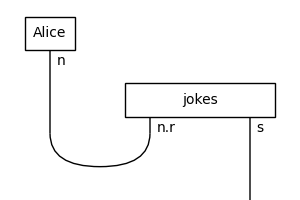ty_factory#

alias of `Ty`

layer_factory#

alias of `Layer`

classmethod cups(left, right)[source]#

Construct a diagram of nested cups for types `left` and `right`.

Parameters:
• left (Ty) – The type left of the cup.

• right (Ty) – Its right adjoint.

Return type:

Diagram

Example

```>>> a, b = Ty('a'), Ty('b')
>>> Diagram.cups(a.l @ b, b.r @ a).draw(figsize=(3, 1),\
... margins=(0.3, 0.05), path='docs/_static/rigid/cups.png')
```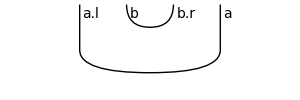classmethod caps(left, right)[source]#

Construct a diagram of nested caps for types `left` and `right`.

Parameters:
• left (Ty) – The type left of the cap.

• right (Ty) – Its left adjoint.

Return type:

Diagram

Example

```>>> a, b = Ty('a'), Ty('b')
>>> Diagram.caps(a.r @ b, b.l @ a).draw(figsize=(3, 1),\
... margins=(0.3, 0.05), path='docs/_static/rigid/caps.png')
```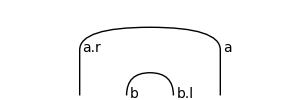curry(n=1, left=True)[source]#

The curry of a rigid diagram is obtained using cups and caps.

```>>> from discopy.drawing import Equation as Eq
>>> x = Ty('x')
>>> g = Box('g', x @ x, x)
>>> Eq(Eq(g.curry(left=False), g, symbol="\$\\mapsfrom\$"),
...     g.curry(), symbol="\$\\mapsto\$").draw(
...         path="docs/_static/rigid/curry.png")
```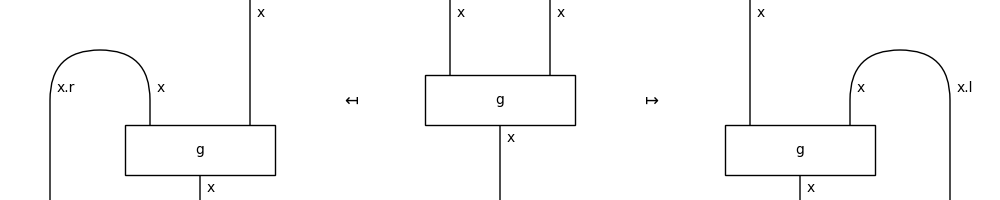Return type:

Diagram

rotate(left=False)[source]#

The half-turn rotation of a diagram, called with `.l` and `.r`.

Example

```>>> from discopy import drawing
>>> x, y = map(Ty, "xy")
>>> f = Box('f', Ty(), x)
>>> g = Box('g', Ty(), x.r @ y)
>>> diagram = f @ g >> Cup(x, x.r) @ y
>>> LHS = drawing.Equation(diagram.l, diagram, symbol="\$\\mapsfrom\$")
>>> RHS = drawing.Equation(LHS, diagram.r, symbol="\$\\mapsto\$")
>>> RHS.draw(figsize=(8, 3), path='docs/_static/rigid/rotate.png')
```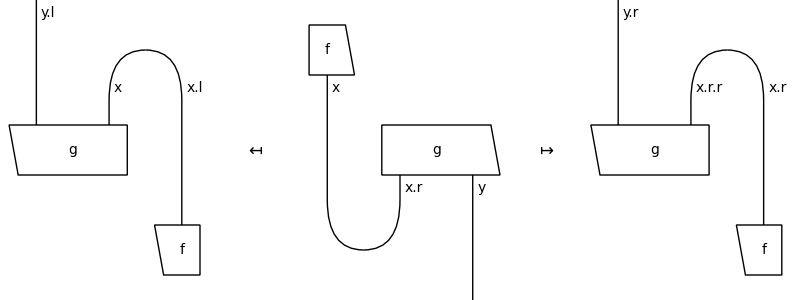transpose(left=False)[source]#

The transpose of a diagram, i.e. its composition with cups and caps.

Parameters:

left – Whether to transpose left or right.

Example

```>>> from discopy.drawing import Equation
>>> x, y = map(Ty, "xy")
>>> f = Box('f', x, y)
>>> LHS = Equation(f.transpose(left=True), f, symbol="\$\\mapsfrom\$")
>>> RHS = Equation(LHS, f.transpose(), symbol="\$\\mapsto\$")
>>> RHS.draw(figsize=(8, 3), path="docs/_static/rigid/transpose.png")
```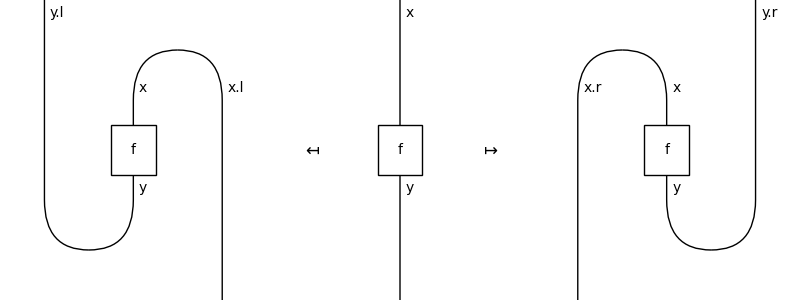transpose_box(i, j=0, left=False)[source]#

Transpose the box at index `i`.

Parameters:
• i – The vertical index of the box to transpose.

• j – The horizontal index of the box to transpose, only needed if the layer `i` has more than one box.

• left – Whether to transpose left or right.

Example

```>>> from discopy.drawing import Equation
>>> x, y, z = Ty(*"xyz")
>>> f, g = Box('f', x, y), Box('g', y, z)
>>> d = (f @ g).foliation()
>>> transpose_l = d.transpose_box(0, 0, left=True)
>>> transpose_r = d.transpose_box(0, 1, left=False)
>>> LHS = Equation(transpose_l, d, symbol="\$\\mapsfrom\$")
>>> RHS = Equation(LHS, transpose_r, symbol="\$\\mapsto\$")
>>> RHS.draw(
...     figsize=(8, 3), path="docs/_static/rigid/transpose_box.png")
```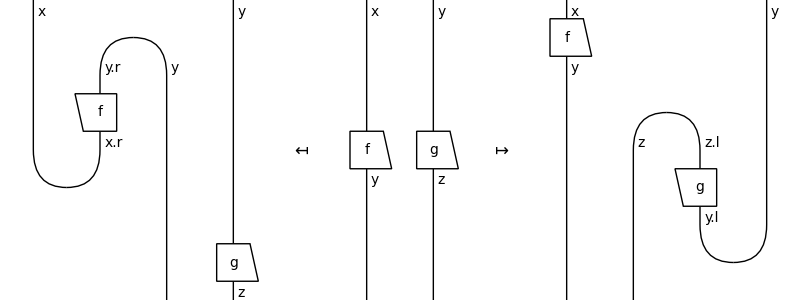snake_removal(left=False)[source]#

Return a generator which yields normalization steps.

Parameters:

left – Passed to `discopy.monoidal.Diagram.normalize()`.

Return type:

Iterator[Diagram]

Example

```>>> from discopy.rigid import *
>>> n, s = Ty('n'), Ty('s')
>>> cup, cap = Cup(n, n.r), Cap(n.r, n)
>>> f, g, h = Box('f', n, n), Box('g', s @ n, n), Box('h', n, n @ s)
>>> diagram = g @ cap >> f[::-1] @ Id(n.r) @ f >> cup @ h
>>> for d in diagram.normalize(): print(d)
g >> f[::-1] >> n @ Cap(n.r, n) >> n @ n.r @ f >> Cup(n, n.r) @ n >> h
g >> f[::-1] >> n @ Cap(n.r, n) >> Cup(n, n.r) @ n >> f >> h
g >> f[::-1] >> f >> h
```
normalize(left=False)#

Return a generator which yields normalization steps.

Parameters:

left – Passed to `discopy.monoidal.Diagram.normalize()`.

Return type:

Iterator[Diagram]

Example

```>>> from discopy.rigid import *
>>> n, s = Ty('n'), Ty('s')
>>> cup, cap = Cup(n, n.r), Cap(n.r, n)
>>> f, g, h = Box('f', n, n), Box('g', s @ n, n), Box('h', n, n @ s)
>>> diagram = g @ cap >> f[::-1] @ Id(n.r) @ f >> cup @ h
>>> for d in diagram.normalize(): print(d)
g >> f[::-1] >> n @ Cap(n.r, n) >> n @ n.r @ f >> Cup(n, n.r) @ n >> h
g >> f[::-1] >> n @ Cap(n.r, n) >> Cup(n, n.r) @ n >> f >> h
g >> f[::-1] >> f >> h
```
normal_form(**params)[source]#

Implements the normalisation of rigid categories, see Dunn and Vicary [DV19], definition 2.12.

Examples

```>>> a, b = Ty('a'), Ty('b')
>>> double_snake = Id(a @ b).transpose()
>>> two_snakes = Id(b).transpose() @ Id(a).transpose()
>>> double_snake == two_snakes
False
>>> *_, two_snakes_nf = monoidal.Diagram.normalize(two_snakes)
>>> assert double_snake == two_snakes_nf
>>> f = Box('f', a, b)
```
```>>> a, b = Ty('a'), Ty('b')
>>> double_snake = Id(a @ b).transpose(left=True)
>>> snakes = Id(b).transpose(left=True) @ Id(a).transpose(left=True)
>>> double_snake == two_snakes
False
>>> *_, two_snakes_nf = monoidal.Diagram.normalize(
...     snakes, left=True)
>>> assert double_snake == two_snakes_nf
```
cap_factory#

alias of `Cap`

cup_factory#

alias of `Cup`

factory#

alias of `Diagram`

sum_factory#

alias of `Sum`